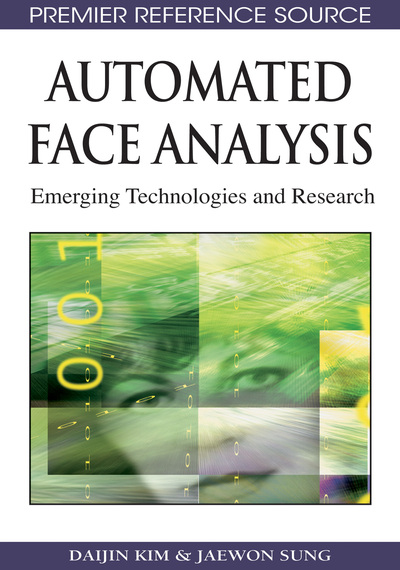# Face Tracking

Daijin Kim (Pohang University of Science & Technology, Korea) and Jaewon Sung (LG Electronics, Korea)
DOI: 10.4018/978-1-60566-216-9.ch004
Available
\$29.50
No Current Special Offers

## Abstract

When we want to analyze the continuous change of the face in an image sequence, applying face tracking methods is a better choice than applying the face detection methods to each image frame. Usually, the face tracking methods are more efficient than the ordinary face detection methods because they can utilize the trajectory of the face in the previous image frames with an assumption that the shape, texture, or motion of the face change smoothly. There have been many approaches to face tracking. We divide the face tracking methods into several categories according to the cues that are extracted for tracking.
Chapter Preview
Top

## 4.1 Particle Filters

For visual object tracking problem, the Kalman filter have been widely used. However, the movement of the visual object does not follow constant velocity or constant acceleration dynamics model. Sometimes, the 2D trajectory of the visual object in an image sequence can change rapidly although the object moves at constant acceleration in the real 3D space. The particle filter is a generic framework that can alleviate this problem. Although the particle filter has been successfully applied to many difficult object tracking problems, the optimal number of partlcles, observation model, and dynamics model should be carefully designed to obtain good results.

The particle filter is a probabilistic approximation algorithm that implements the Bayesian filter, where they try to estimate the state of a dynamic system from a noisy observation probabilistically. Figure 1 illustrates a graphical model of the Bayesian filters, where xt and yt are the hidden variable and the observed variable, respectively.

Bayesian filters take three important assumptions such as (i) the conditional independence, (ii) probabilistic relationship like Gaussian model, and (iii) the first order Markov process. Also, they include the motion modelthat is used to update the temporal change of the motion stateand the sensor likelihood modelthat is used to update the measurement weights.

When we denote an observation sequence from time index 1 to t as=(y1,y1,…,yt), the operating process of the Bayesian filters can be described by the following two equations as

(4.1.1) (4.1.2)

Here, Eq. (4.1.1) and (4.1.2) represent the time update that predicts the next state by the motion model, and the measurement update that predicts the next observation by the sensor likelihood model, respectively. According to Bayes rule, the posterior distributioncan be computed by the product of the prior distributionand the sensor likelihood model, where the evidenceis used as a normalization factor.

Among several Bayesian filter, the Kalman filter and the particle filter are generally used for the location tracking. The Kalman filter is an optimal estimator, where it assumes that the random variable is the Gaussian model and both the motion model and the sensor likelihood model are linear functions of the states. However, the particle filter can represent arbitrary probability densities. It can converge to the true posterior even in the non-Gaussian model and nonlinear dynamic systems

## Complete Chapter List

Search this Book:
Reset